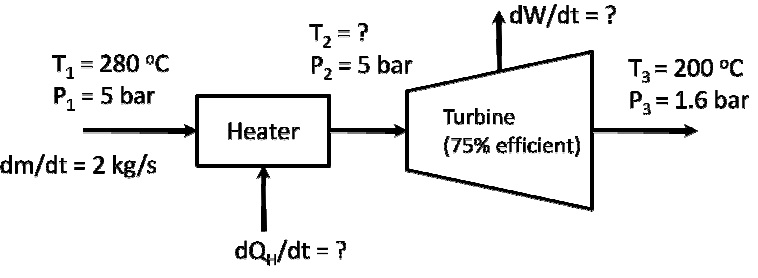Problem on heat of sublimation

Using the vapor pressure data provided below, estimate

i) the heat of sublimation of ice,

ii) the heat of vaporization of water,

iii) the heat of fusion of ice and compare your estimate with the published value of 6010 J/moL

iv) the triple point of water (pressure and temperature). Solve the nonlinear.

T(°C)                             Vapor Pressure (mm Hg)
Ice                                       -4                                            3.280
-2                                            3.880
Water                                   +2                                            5.294
+4                                            6.101

E

Expert

##### Verified

At 1 atm pressure, ice melts at 0°C

density of ice = 920 kg/m3
density of liquid water = 997 kg/m3

Now using the Clapeyron equation

dP/dT = LP/RT2  [ where Vg>>>Vl]

On integration:

Ln[P1/P2] = -L/R[ 1/T1 -1/T2]

Ln(3.880/3.280)= -L/R [ 1/271-1/269]

So Ice  L = ΔH sublimation = - 50911.5 J/mol =-50.9115KJ/mole

Similarly for water L = ΔH vap =- 44929.9 J/Mole =- 44.929 KJ/mole

Now At triple point Ice /Water /Saturate water Vap co-exists Now using the Thermo concept at triple point

ΔH sublimation = ΔH vap + ?H fusion

Similarly  at triple vap pressure of water = vap pressure of ice

LnP = ln(3.880)-50911.5/8.3145[1/T – 1/271] = ln(5.294)-44929.9/8.3145[1/T-1/275]

So Solving for T we get T =273.1297K

Now Solving for P we get

LnP = ln(3.880)-50911.5/8.3145[1/273.129 – 1/271]
LnP = 1.532014

So P = 3.589546 mmHg

#### Related Questions in Chemical Engineering

• ##### Q :Rate of flow of nitrogen The feed to an

The feed to an ammonia synthesis reactor contain 25 mole% nitrogen and the balance hydrogen. The flow rate of the stream is 3000 kg/hr. Calculate the rate of flow of nitrogen into the reactor in kg/hr. (Hint: First calculate the average molecular weight of the mixture).

• ##### Q :Problem on weight fraction A gas

A gas contains 350 ppm of H2S in CO2 at 72°F and 1.53 atm pressure. If the gas is liquified, what is the weight fraction H2S?

• ##### Q :Problem on molar flow rate I) Sulphur

I) Sulphur dioxide (SO2) with a volumetric flow rate 5000cm3/s at 1 bar and 1000C is mixed with a second SO2 stream flowing at 2500cm3/s at 2 bar and 200C. The process occurs at steady state. You may as

• ##### Q :I need to solve the material and energy

I need to solve the material and energy balance for the att.

• ##### Q :Problem on entropy A heater (heat

A heater (heat source temperature = 527 K) and turbine are connected in series as shown below:##### Q :Draw a flow diagram and annotate the

Cumene is produced by the vapour phase reaction of benzene with propylene. The fresh feeds have the following compositions:

Benzene: 99% w/w benzene, 1% w/w toluene

Propylene: 98% v/v propylene,

• ##### Q :Hh to determine the temperature that

to determine the temperature that occurred in a fire in a warehouse, the arson investigator noticed that the relief valve on a methane storage tank had popped open at 3000 psig, the rated value. Before the fire started, the tank was presumably at ambient conditions and the gage read 1950 psig. If th

• ##### Q :Problem on heat of sublimation Using

Using the vapor pressure data provided below, estimate

i) the heat of sublimation of ice,

ii) the heat of vaporization of water,

iii) the heat of fusion of ice and compare your estimate with the published value of 6010 J/moL

iv) the triple point of wate

• ##### Q :Temprature To determine the temperature

To determine the temperature that occurred in a ?re in a warehouse, the arson investigator noticed that the relief valve on a methane storage tank had popped open at 3000 psig, the rated value. Before the ?re started, the tank was presumably at ambient conditions and the gage read 1950 psig. If

• ##### Q :Materials and energy balance you look

you look up the specific gravity of 96% H2SO4 and find it is 1.858.calculate the weight